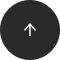SQLite

# How to Get the Current Time in SQLite

#### Operators:

TIME('now'), TIME()

#### Problem:

You’d like to get the current time in an SQLite database.

#### Solution:

We’ll use the function `TIME()` to get the current time.

```SELECT TIME(‘now’);
```

Here’s the result of the query:

`10:56:12`

#### Discussion:

The SQLite function `TIME()` returns the current time as a text value. It uses the 'hh:mm:ss' format, where hh is a 2-digit hour, mm is a 2-digit minute, and ss is a 2-digit second.

This function has only one argument: the text value ‘now’, which indicates that the current date and time should be returned. You can omit this argument, as it is the default. Here is the shorter version of this function:

```SELECT TIME();
```

#### Recommended articles: